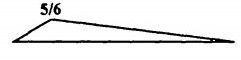top of pageSearch

# Structural Analysis (121-140)

Updated: Apr 30, 2020

121.The influence line diagram for force in member "XY" of the truss shown in Fig.

will be122.A truss is shown in Fig. The cross sectional area of each member is 'A' and

the modulus of elasticity of the material is E. The strain energy in the member XY is given byzero

123.A rigid cantilever frame ABC is fixed at C. It carries a load P at A, as shown in

Fig. Neglecting axial deformation, the vertical deflection of the point A is

given by

Pl²(2h+l)/2EI

124. The horizontal thrust due to rise in temperature in a semi-circular two hinged

arch of radius R is proportional to 1/R²

125.For the continuous beam (EI constant) loaded as shown in Fig. the

moment at 'B' is

0.75 times free moment at mid-span

126. A fixed beam AB of length ' l ' having constant flexural rigidity EI carries two

loads P at its third points C and D as shown in Fig.Numerically, maximum bending moment will occur

at A and at B and will be equal to 2P//9

127. Consider the following statements: Sinking of an intermediate support of a

continuous beam

1. reduces the negative moment at support.

2. increases the positive moment at the centre of span.

128. A number of wheel loads 3t, 4t, 5t and 6t spaced 2 m, 3m and 4m respectively move on a simply supported beam AB of span 24 m with the 3t load leading from left to right. To find the maximum bending moment at 18 m from A, the load that

must be placed at the section is 4t

129. A load ' W' is moving from left to right support on a simply supported beam of

span T . The maximum bending moment at 0.4 1 from the left support is 0.24 Wl

shown in Fig. 12.46 and 12.471. (1/EI) x BM at B of beam in Fig. 12.47.

2.(1/EI) x moment of the area about B of BMD of beam in Fig. 12.46.

131.Which of the following pairs of a given beam and its conjugate beam are correctly matched ?132. A cantilever beam AB, fixed at A and carrying a load W at the free end B, is

found to deflect by 8 at the mid-point of AB. The deflection of B due to a load W/2

at the mid-point will b 2δ

133.The frame shown in Fig. is subjected to loads as indicated. The

intensity of lateral load is 2 kN/m. The shape of the bending moment diagram for

this frame will be134. In the frame shown in Fig. 12.51, support 4 settles by 8.The fixed end moment in the horizontal member of the frame will be (other

symbols have the usual meaning) 6EIδ/16

135.A plane frame is loaded as shown in Fig. 12.52. The rotations are indicated as θʙ and θᴄ and the sway is indicated by the symbol Δθʙ = - θᴄ ; Δ is present

136.For the plane frame shown in Fig. 12.53, static and kinematic degrees of

indeterminacy are respectively9 and 24

137. A truss of panel dimensions 3 m x 4 m is shown in figThe influence line diagram for the force in the member UL is138. What is the horizontal deflection at free end C of the frame shown in Fig. ?Ml²/EI

139.Which one of the following gives the distribution factors for members AE and

AC of box section shown in Fig. ? 0.5 and 0.5

140.For the fixed beam shown in Fig. 12.58, the influence line diagram for the bending

moment at the mid-span of the beam is best represented as